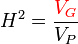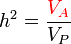# HeritabilityThis article was checked by pedagogue, but more than year ago. Signature:  OSeda (talk)

 English: HeritabilityCzech: Heritabilita, dědivostHeritability denotes the fraction of trait variance due to genetic factors (VG) over the total trait variance (VP). This broad-sense heritability H2 is thus calculated as follows:$H^2 = \frac{\color{Red}V_G}{V_P}$

H2 can theoretically reach values between 0 and 1; if H2 = 0, the trait/phenotype variance is fully dependent on environmental factors, on the contrary, if H2 = 1 the environmental factors play no role and the trait variation is determined only genetically.

Often we need to know just the share of additive genetic component (VA) responsible for trait variance, i.e. the narrow-sense heritability, dubbed h2 and calculated as follows$h^2 = \frac{\color{Red}V_A}{V_P}$